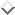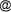## Nurhayati, Grete Thinggaard, Udo ter Meulen: Accumulation of $15$N in Yolks and Albumen of Hens Fed Diets Containing $15$N-CCC During Egg Development

NURHAYATI$1$, GRETE THINGGAARD$2$, UDO TER MEULEN$2$
$1$University of Jambi, Department of Animal Nutrition and Feed Science, Indonesia
$2$Georg-August-Universität Göttingen, Institute of Animal Physiology and Nutrition, Germany

An experiment was conducted to evaluate the accumulation of dietary $15$N-CCC in yolks and albumen of hens fed diets containing $15$N-CCC during egg development. Twenty 280 days old Brown breed layer hens were fed a $15$N-CCC free balanced diet with 16.37% crude protein and 11.48 MJ ME/kg. In completely randomised design, the hens were divided into 4 groups (A,B,C and D) and given on one of the 4 treatments: 0 mg $15$N-CCC/kg feed (control diet/group A), 5 mg $15$N-CCC/kg feed (group B), 50 mg $15$N-CCC/kg feed (group C) and 100 ppm mg $15$N-CCC/kg feed (group D) for 11 days. During the 7 days followed, $15$N-CCC treatments were withdrawn and all chickens restored to feeding on the control diet. Eggs were collected daily during both periods and egg yolks and albumen were separated. The $15$N content was measured using a coupled Elemental Analyser-Continuous Flow II Interface-Isotope Ratio Mass Spectrometer (EA-ConFloII Interface-IRMS) and the $δ15$N excess ( $δ15$N-ex) and atom percentage $15$N (At%) calculated. There was no significant ($p > 0.05$) difference in $δ15$N-ex and At% of egg yolks and albumen of group B both during 11 days of feeding on $15$N-CCC containing diets and during the 7 days $15$N" CCC diets withdrawal. Feeding with $15$N" CCC affected ($p < 0.05$) $δ15$N-ex and At% in egg yolks and albumen of group C and D from 8 and 3 days respectively after the beginning of feeding $15$N-CCC diets, and up to 2 and 4 days after $15$N" CCC diets were withdrawn, respectively. The $δ15$N-ex and At% in egg yolks tended to be higher than in albumen during the treatment period. The $15$N concentrations reduced after $15$N-CCC diets were withdrawn. These results suggest that hens might transfer excess dietary $15$N-CCC or its metabolites into eggs and $15$N accumulates during egg development. However, by this method it is not possible to determine if $15$N is still bound in CCC or in its metabolite products.

Keywords: Atom percentage $15$N, chlorocholine chloride, $δ15$N, egg

#### Footnotes

...PContact Address: Udo ter Meulen, Georg-August-Universität Göttingen, Institute of Animal Physiology and Nutrition, Kellnerweg 6, 37077 Göttingen, Germany, e-mail: umeulengwdg.de
Andreas Deininger, 2003